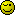# Tree traversal/recursion

I have a tree structure in the format:

``````Array
(
 => TEST
 => Array
(
 => TEST
 => number
 => 4321
)

 => Array
(
 => TEST
 => Array
(
 => TEST
 => Array
(
 => TEST
 => part
 =>
)

 => Array
(
 => TEST
 => number
 => 1234
)
)
)
)
``````

I am trying ot traverse the thing and add a root node to each non-leaf node so no matter where I am in the tree I can reverse backwards if needed.

I have this code but for the life of me I cannot figure out why the arrays are not referenceing. I am certain I have done this in the past as I remember referenced nodes dump as recursion or something similar but again obviously my code is not properly referencing nodes as entire copies are insrted instead of the recursion I was hoping for.

``````function tree_make_bidirectional(&\$elements)
{
\$temp = array();

\$temp['ROOT'] = &\$elements;

foreach(\$elements as &\$element){
if(is_array(\$element)){
\$temp[] = tree_make_bidirectional(\$element);
}
else{
\$temp[] = &\$element;
}
}

return \$temp;
}
``````

Anyone spot the issue and care to point it out?

Cheers,
Alex

I’m also not 100% sure what you’re trying to do, but if you want to use arrays as trees then best to make them a balanced binary tree. If you do that, left and right child are always n2 and n2+1, likewise, parents are n/2.

I don’t quite understand what you’re trying to do. Maybe if you could give an example of how the array is supposed to become?

I didn’t figure it out actually, thought I did, spoke to soon.

I’ve modified the code several times resulting in this:

[syntax=php] function tree_make_bidirectional(&\$nodes, &\$root = null)
{
foreach(\$nodes as &\$node){
if(is_array(\$node)){
tree_make_bidirectional(\$node, \$nodes);
}
}

``````\$nodes['ROOT'] = &\$root;

return \$nodes;
``````

}[/syntax]

This results in a mild explosion of tree data being duplicated…

[syntax=php] function tree_make_bidirectional(&\$nodes, &\$root = null)
{
static \$depth = 0;

``````\$nodes['ROOT'] = \$depth;

foreach(\$nodes as &\$node){
if(is_array(\$node)){
\$depth++;
tree_make_bidirectional(\$node);
\$depth--;
}
}

if(\$nodes['ROOT'] === 0){
\$nodes['ROOT'] = null;
}
else{
\$nodes['ROOT'] = &\$nodes;
}

return \$nodes;
``````

}[/syntax]

This snippet almost works though the references are pointing to themselves…I need ROOT to point to one item above that.

Cheers,
Alex

I figured it out, but essentially take the first array and each node should have a ROOT element added that can be used to reference the parent node.

It’s easy to traverse forward in a tree structure but reverse requires a root node element, which is what I was after.

Cheers,
Alex

I’m glad you figured it outWould you mind posting the solution here, so others (me) can learn from it?